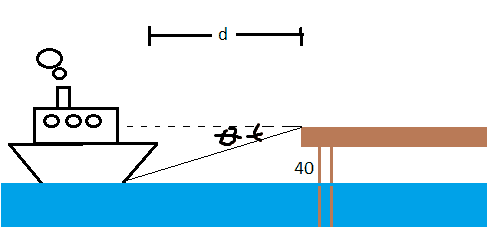# Trigonometry Word Problem involving Inverse trigonometric functions

acen_gr

## Homework Statement

A ship is d feet from a dock (horizontal distance). The dock is 40 feet above sea level. The angle of depression from the dock to the ship is θ. Write θ as a function of d.

## Homework Equations

This question is accompanied with choices. The choices are
$$(A) θ = 40arctan(d)$$
$$(B) θ = arctan(\frac{40}{d})$$
$$(C) θ = arcsin(\frac{d}{60})$$
$$(D) θ = \frac{arctan(d)}{40}$$
$$(E) none of these$$

## The Attempt at a Solution

First, I would like to know if my graphical representation of this problem is correct.Thank you!

Last edited:

Homework Helper
Do you know the definitions of "sine", "tangent", etc.? If not, look them up. Once you know them you will see that this problem is trivial.

Homework Helper
It is a very nice (and correct) drawing, with a very big ship.ehild

acen_gr
Do you know the definitions of "sine", "tangent", etc.? If not, look them up. Once you know them you will see that this problem is trivial.
It is a very nice (and correct) drawing, with a very big ship.ehild

Ok sir so if my interpretation of the problem is right, then it would be right if I answer choice B right? Thank you!Homework Helper
Ok sir so if my interpretation of the problem is right, then it would be right if I answer choice B right? Thank you!YES!

ehild

acen_gr
YES!

ehild

Yes! I made it right. I just wonder if my understanding was right because I'm not from an English-based country so some phrasing are not clear to me. Thank you everyone!